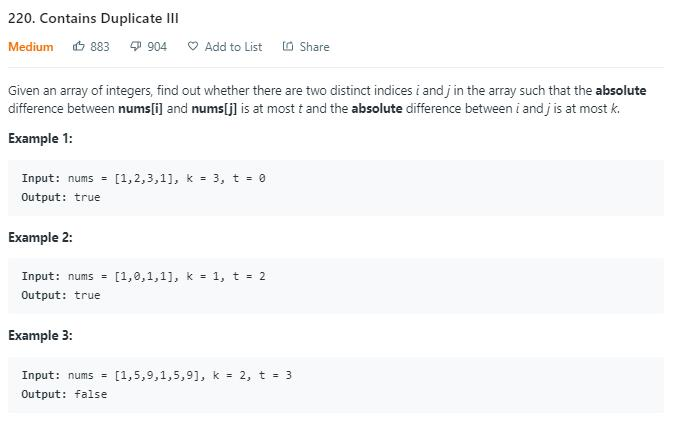# 题目描述（中等难度）# 解法一 暴力

public boolean containsNearbyAlmostDuplicate(int[] nums, int k, int t) {
int n = nums.length;
for (int i = 0; i < n; i++) {
for (int j = 1; j <= k; j++) {
if (i + j >= n) {
break;
}
if (Math.abs(nums[i] - nums[i + j]) <= t) {
return true;
}
}
}
return false;
}


public boolean containsNearbyAlmostDuplicate(int[] nums, int k, int t) {
int n = nums.length;
for (int i = 0; i < n; i++) {
for (int j = 1; j <= k; j++) {
if (i + j >= n) {
break;
}
if (Math.abs((long) nums[i] - nums[i + j]) <= t) {
return true;
}
}
}
return false;
}


• 正数减正数，不会产生溢出。

• 正数减负数，结果一定是正数，如果此时结果是负数，说明出现了溢出。

• 负数减正数，结果一定是负数，如果此时结果是正数，说明出现了溢出。这里还有一种特殊情况需要考虑，我们知道负数比正数多一个。最小的负数是 -2147483648，最大的正数是 2147483647

如果我们计算 -2147483647 - 1 = -2147483648，虽然结果没有溢出，但如果取绝对值，由于正数不能表示 2147483648 ，所以这种情况也需要看成溢出。

• 负数减负数，不会产生溢出。

public boolean containsNearbyAlmostDuplicate(int[] nums, int k, int t) {
int n = nums.length;
for (int i = 0; i < n; i++) {
for (int j = 1; j <= k; j++) {
if (i + j >= n) {
break;
}
int sub = nums[i] - nums[i + j];
//正数减负数, 0 算作正数，因为 0 - (-2147483648) 会出现溢出
if (nums[i] >= 0 && nums[i + j] < 0) {
if (sub < 0) {
continue;
}
}
//负数减正数
if (nums[i] < 0 && nums[i + j] >= 0) {
if (sub > 0 || sub == Integer.MIN_VALUE) {
continue;
}
}
if (Math.abs(sub) <= t) {
return true;
}
}
}
return false;
}


# 解法二

k = 3,  t = 2, 窗口内 3 个数，当前考虑 x
2 6 3 x 5
^   ^

2 6 3 x 5
^   ^



public boolean containsNearbyAlmostDuplicate(int[] nums, int k, int t) {
//为了得到窗口内的最大数
TreeMap<Integer, Integer> maxTreeMap = new TreeMap<>(new Comparator<Integer>() {
@Override
public int compare(Integer i1, Integer i2) {
return i2 - i1;
}
});
//为了得到窗口内的最小数
TreeMap<Integer, Integer> minTreeMap = new TreeMap<>();
int n = nums.length;
if (n == 0) {
return false;
}
maxTreeMap.put(nums, 1);
minTreeMap.put(nums, 1);
for (int i = 1; i < n; i++) {
//将窗口内的第一个数删除
if (i > k) {
remove(maxTreeMap, nums[i - k - 1]);
remove(minTreeMap, nums[i - k - 1]);
}
if (maxTreeMap.size() == 0) {
continue;
}
long max = maxTreeMap.firstKey();
long min = minTreeMap.firstKey();
//和最大数以及最小数进行比较
if (nums[i] >= max) {
if (nums[i] - max <= t) {
return true;
}
} else if (nums[i] <= min) {
if (min - nums[i] <= t) {
return true;
}
} else {
for (int j = 1; j <= k; j++) {
if (i - j < 0) {
break;
}
if (Math.abs((long) nums[i] - nums[i - j]) <= t) {
return true;
}
}
}
//当前数加入窗口
}
return false;
}

private void add(TreeMap<Integer, Integer> treeMap, int num) {
// TODO Auto-generated method stub
Integer v = treeMap.get(num);
if (v == null) {
treeMap.put(num, 1);
} else {
treeMap.put(num, v + 1);
}
}

private void remove(TreeMap<Integer, Integer> treeMap, int num) {
// TODO Auto-generated method stub
Integer v = treeMap.get(num);
if (v == 1) {
treeMap.remove(num);
} else {
treeMap.put(num, v - 1);
}
}


# 解法三 set

k = 3,  t = 2, 窗口内 3 个数用 TreeSet 存储, 当前考虑 x
2 6 3 x 5
^   ^


public boolean containsNearbyAlmostDuplicate(int[] nums, int k, int t) {
TreeSet<Long> set = new TreeSet<>();
int n = nums.length;
for (int i = 0; i < n; i++) {
if (i > k) {
set.remove((long)nums[i - k - 1]);
}
Long low = set.ceiling((long) nums[i] - t);
//是否找到了符合条件的数
if (low != null && low <= (long)nums[i] + t) {
return true;
}
}
return false;
}


# 解法四 map

t = 2, 每个桶内的数字范围如下

-------        -------       -------     -------

-------        -------       -------     -------


k = 3,  t = 2, 窗口内 3 个数用上边的桶存储, 当前考虑 x
2 6 3 x 5
^   ^

0             1              2
-------        -------       -------
|     2  |      |  3    |     |   6   |
-------        -------       -------


//w 表示桶中的存储数字范围的个数
private long getId(long num, long w) {
if (num >= 0) {
return num / w;
} else {
//num 加 1, 把负数移动到从 0 开始, 这样算出来标号最小是 0, 已经用过了, 所以要再减 1
return (num + 1) / w - 1;
}
}


「桶」放到代码中我们要什么数据结构存储呢？我们注意到，桶中其实最多就只会有一个数字（如果有两个数字，说明我们已经找到了相差小于等于 t 的数，直接结束）。所以我们完全可以用一个 mapkey 表示桶编号，value 表示桶中当前的数字。

public boolean containsNearbyAlmostDuplicate(int[] nums, int k, int t) {
if (t < 0) {
return false;
}
HashMap<Long, Long> map = new HashMap<>();
int n = nums.length;
long w = t + 1; // 一个桶里边数字范围的个数是 t + 1
for (int i = 0; i < n; i++) {
//删除窗口中第一个数字
if (i > k) {
map.remove(getId(nums[i - k - 1], w));
}
//得到当前数的桶编号
long id = getId(nums[i], w);
if (map.containsKey(id)) {
return true;
}
if (map.containsKey(id + 1) && map.get(id + 1) - nums[i] < w) {
return true;
}

if (map.containsKey(id - 1) && nums[i] - map.get(id - 1) < w) {
return true;
}
map.put(id, (long) nums[i]);
}
return false;
}

private long getId(long num, long w) {
if (num >= 0) {
return num / w;
} else {
return (num + 1) / w - 1;
}
}